# GMAT Math : DSQ: Calculating the area of a rectangle

## Example Questions

### Example Question #71 : Quadrilaterals

Data sufficiency question- do not actually solve the question

Does the square or rectangle have a greater area?

1. The perimeter of both the square and rectangle are equal.

2. The rectangle does not have four equal sides.

Statement 1 is sufficient, but statement 2 is not sufficient to answer the question

Each statement is sufficient

Statement 2 is sufficient, but statement 1 is not sufficient to answer the question

Both statements taken together are sufficient to answer the question, but neither statement alone is sufficient.

Both statements taken together are sufficient to answer the question, but neither statement alone is sufficient.

Explanation:

When a square and rectangle have the same perimeter, the square will have a larger area because having 4 equal sides maximizes the area. However, from statement 1, it is impossible to tell if the rectangle is also a square. When the information from statement 2 is combined, we can conclude that the rectangle is not also a square.

### Example Question #115 : Geometry

What is the area of a rectangle?

Statement 1: The length of its diagonal is 25.

Statement 2: The diagonal and either of its longer sides form a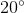angle.

BOTH statements TOGETHER are sufficient to answer the question, but NEITHER statement ALONE is sufficient to answer the question.

EITHER statement ALONE is sufficient to answer the question.

Statement 2 ALONE is sufficient to answer the question, but Statement 1 ALONE is NOT sufficient to answer the question.

Statement 1 ALONE is sufficient to answer the question, but Statement 2 ALONE is NOT sufficient to answer the question.

BOTH statements TOGETHER are insufficient to answer the question.

BOTH statements TOGETHER are sufficient to answer the question, but NEITHER statement ALONE is sufficient to answer the question.

Explanation:

To find the rectangle, you need the length and the width.

If you know the diagonaland the angleit forms with one of the longer sides, you can use trigonometry to find both length and width: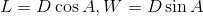From there, the area follows.

If you know only the diagonal, you have insufficient information; the length and width can vary according to that angle. If you only know the angle, you can discern the proportions of the sides, but not the actual lengths.

### Example Question #116 : Geometry

A rectangle has vertices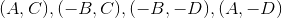where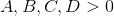Of the four quadrants, which one includes the greatest portion of the rectangle?

Statement 1: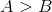Statement 2: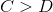Statement 1 ALONE is sufficient to answer the question, but Statement 2 ALONE is NOT sufficient to answer the question.

BOTH statements TOGETHER are insufficient to answer the question.

BOTH statements TOGETHER are sufficient to answer the question, but NEITHER statement ALONE is sufficient to answer the question.

Statement 2 ALONE is sufficient to answer the question, but Statement 1 ALONE is NOT sufficient to answer the question.

EITHER statement ALONE is sufficient to answer the question.

BOTH statements TOGETHER are sufficient to answer the question, but NEITHER statement ALONE is sufficient to answer the question.

Explanation:

The portion of the rectangle to the right of the-axis has area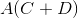; to the left,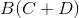. From Statement 1 alone, since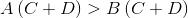, and the portion of the rectangle on the right is greater than the portion on the left. However, this is all we can determine.

By a similar argument, from Statement 2 alone, the portion of the rectangle above is greater than the portion below, but this is all we can determine.

From both statements together, however, we can compare the portions of the rectangles in the four quadrants. The areas of each are:

Quadrant I: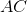Quadrant II: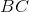Quadrant III: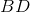Quadrant IV: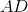Sinceandis the greatest of the four quantities, and we see that Quadrant I includes the lion's share of the rectangle.

### Example Question #117 : Geometry

Rectangleis inscribed in a circle. What is its area?

Statement 1; The circle has area.

Statement 2: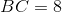BOTH statements TOGETHER are insufficient to answer the question.

BOTH statements TOGETHER are sufficient to answer the question, but NEITHER statement ALONE is sufficient to answer the question.

Statement 1 ALONE is sufficient to answer the question, but Statement 2 ALONE is NOT sufficient to answer the question.

EITHER statement ALONE is sufficient to answer the question.

Statement 2 ALONE is sufficient to answer the question, but Statement 1 ALONE is NOT sufficient to answer the question.

BOTH statements TOGETHER are sufficient to answer the question, but NEITHER statement ALONE is sufficient to answer the question.

Explanation:

The figure referenced is below: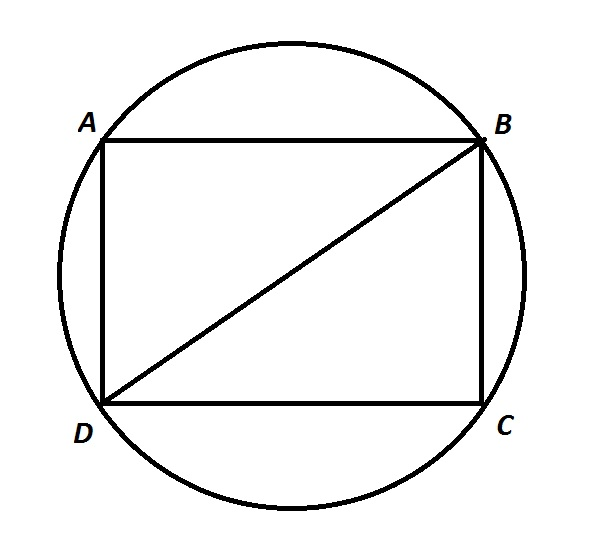Assume Statement 1 alone.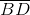is a diagonal of the rectangle, and also a diameter of the circle. Statement 1 gives the area of the circle, from which the radius, and, subsequently,, can be calculated. However, infinitely many rectangles of different areas can be constructed in this circle, so without any further information, it is not clear what the sidelengths are - and what the area is.

Assume Statement 2 alone. This statement only gives the length of one side. Without any further information, the area of the rectangle is unknown.

Now assume both statements are true.can be calculated, andis given, so the Pythagorean Theorem can be used to find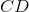. The area of the rectangle is the product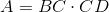, so the two statements together are sufficient.

### Example Question #118 : Geometry

Rectangleis inscribed in a circle. What is the area of the rectangle?

Statement 1: The circle has area 77.

Statement 2: The rectangle is a square.

Statement 2 ALONE is sufficient to answer the question, but Statement 1 ALONE is NOT sufficient to answer the question.

BOTH statements TOGETHER are sufficient to answer the question, but NEITHER statement ALONE is sufficient to answer the question.

BOTH statements TOGETHER are insufficient to answer the question.

Statement 1 ALONE is sufficient to answer the question, but Statement 2 ALONE is NOT sufficient to answer the question.

EITHER statement ALONE is sufficient to answer the question.

BOTH statements TOGETHER are sufficient to answer the question, but NEITHER statement ALONE is sufficient to answer the question.

Explanation:

The figure referenced is below (note that the figure itself assumes Statement 2, but this is not known from Statement 1):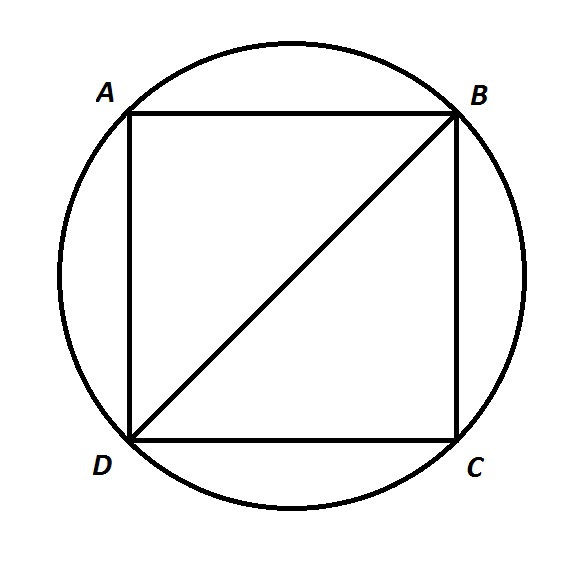Assume Statement 1 alone. A diagonal of a rectangle inscribed inside a circle is a diameter of the circle. Statement 1 gives the area of the circle, from which the radius, and, subsequently,, can be calculated. However, infinitely many rectangles of different areas can be constructed in a given circle, so without any further information, it is not clear what the sidelengths are - and what the area is.

Assume Statement 2 alone. It follows that all of the sides of the rectangle/square are congruent, but without the common sidelength, the area of the square cannot be calculated.

Assume both statements.can be calculated, and the area of the square can be calculated to be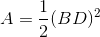.

### Example Question #111 : Geometry

Give the area of a given rectangle.

Statement 1: The perimeter of the rectangle is 36.

Statement 2: All sides of the rectangle have a length equal to an odd prime integer.

EITHER statement ALONE is sufficient to answer the question.

BOTH statements TOGETHER are insufficient to answer the question.

BOTH statements TOGETHER are sufficient to answer the question, but NEITHER statement ALONE is sufficient to answer the question.

Statement 2 ALONE is sufficient to answer the question, but Statement 1 ALONE is NOT sufficient to answer the question.

Statement 1 ALONE is sufficient to answer the question, but Statement 2 ALONE is NOT sufficient to answer the question.

BOTH statements TOGETHER are insufficient to answer the question.

Explanation:

Assume both statements. Then from Statement 1, it follows that: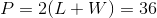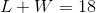There are two pairs of odd primes that add up to 18 - (5,13), in which case the area is 65, and (7,11), in which case the area is 77. The two statements together are inconclusive.

### Example Question #120 : Geometry

Give the area of a given rectangle.

Statement 1: The perimeter of the rectangle is 10.

Statement 2: All sides of the rectangle have a length equal to a prime integer.

Statement 2 ALONE is sufficient to answer the question, but Statement 1 ALONE is NOT sufficient to answer the question.

Statement 1 ALONE is sufficient to answer the question, but Statement 2 ALONE is NOT sufficient to answer the question.

EITHER statement ALONE is sufficient to answer the question.

BOTH statements TOGETHER are sufficient to answer the question, but NEITHER statement ALONE is sufficient to answer the question.

BOTH statements TOGETHER are insufficient to answer the question.

BOTH statements TOGETHER are sufficient to answer the question, but NEITHER statement ALONE is sufficient to answer the question.

Explanation:

A a rectangle with sides of length 1 and 4 and a rectangle with sides of length 2 and 3 both have perimeter 10, but they have different areas ( 4 and 6, respectively), making Statement 1 alone inconclusive. Statement 2 is inconclusive, there being infinitely many primes.

Assume both statements.

Then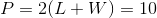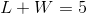Sinceand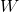are both prime integers, one must be 2 and the other must be 3 (1 and 4 cannot be a possibility, since 1 is not a prime). It does not matter which is which, so the numbers can be multiplied to obtain area 6.

Tired of practice problems?

Try live online GMAT Math prep today.